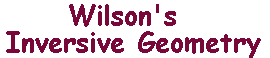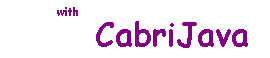The object of these pages is to introduce inversive geometry. Many of the results and ideas are Greek, largely due to Apollonius of Perga We shall approach from the Klein viewpoint, that is to say using a group of transformations of a set of points. To motivate the definitions of the set and its transformations, we begin by looking at a classical greek Theorem (Apollonius's Theorem). Whenever it is useful, we give CabriJava (interactive) illustrations. For example, the CabriJava pane on the right shows three touching red circles. The blue and green circles each touch all of the red circles. By dragging A or B, you can change the red circles, but it is always possible to draw the blue and green circles. Why? That's what inversive geometry is about. You can find an inversive proof here. table of contents
related pages

appendices
main geometry page